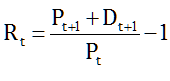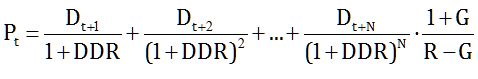Security analysis cannot presume to lay down general rules as to the “proper value” of any given common stock. Practically speaking, there is no such thing”.

I hadn’t yet read Ben Graham when, many years ago, I began working as a portfolio manager for a large global asset management company. Their investment process was heavily reliant on the input of security analysts. Each of them followed a number of companies in a certain sector. Their task was to calculate the “proper value” of each stock and condense it into a Dividend Discount Rate.

A stock price is, by definition, the discounted sum of expected future dividends. If Pt is today’s price and Pt+1 is the price at some future date (say in a year), then the total return over the period is:which is the sum of a capital gain or loss (Pt+1-Pt)/Pt deriving from the increase or decrease in the stock price, and a dividend yield Dt+1/Pt deriving from a dividend D that the company may distribute at some point over the period. Reordering the definition above, we have Pt=(Pt+1+Dt+1)/(1+Rt) and therefore Pt+1=(Pt+2+Dt+2)/(1+Rt+1). Hence, by forward substitution:We can keep replacing P forward N times, but at some point we need to stop and assume that, from that point onwards, dividends will grow at a constant rate G and will be discounted at a constant rate R:If we call S the term in square brackets, multiply it by (1+G)/(1+R) and subtract it from S, we get S=(1+G)/(R-G). Therefore, the terminal value Pt+N equals Dt+N(1+G)/(R-G) and:Given the stream of dividends from t to t+N, a terminal growth rate and a terminal discount rate, the Dividend Discount Rate is the Internal Rate of Return that equates the expression above to the current market price of the stock:The lower the market price, the higher the DDR. Other than that, the DDR depends on analyst inputs: positively on the stream of dividends and the terminal growth rate, and negatively on the terminal discount rate.

Virtually all of the analyst’s work is focused on forecasting the dividend stream. As dividends come out of earnings, analysts acquire a wealth of knowledge about the company and the industry in which it competes, and develop highly elaborate models with the purpose of predicting what earnings will be, not just this year or next, but far out in the future. Then, to close the model, they pick a number for the terminal growth rate – usually the “growth rate of the economy”: a company cannot grow forever at a higher rate – and another, larger number for the terminal discount rate, also known as the cost of capital, reflecting, in all sorts of often fanciful ways, the company’s “riskiness”.

As an example, let’s take a stock with a market price of 100, and let’s say that, after diligent and painstaking work, the analyst has come up with the following stream of dividends for the next ten years: (2, 4, 4, 4, 5, 5, 5, 6, 6, 7). He then assumed G=3% and R=5%. Result: DDR=16.1%. The DDR is quite sensitive to the market price: If the price drops by 10%, the DDR goes up to 17.5%; if the price goes up by 10%, the DDR drops to 14.9%. But how sensitive is it to analyst inputs? Imagine for example that the company goes through tough times and, as a result, the analyst expects it to lose money and cut dividends to zero for the next three years. Alternatively, imagine that for some reason – perhaps the stock’s beta has changed – the analyst decides to nudge up the terminal discount rate from 5% to 5.25%. Which change has the larger impact of the DDR? Clearly a loaded question: the earnings cataclysm reduces the DDR to 15.2%; the beta tweak to 14.9%. You can play with the numbers here.

Does this mean that DDR modelling is wrong or useless? No. Stock prices are the discounted sum of expected cash flows made available to shareholders, and cash flow modelling is the right framework to think about equity valuations, to which many other fancier and convoluted alternatives can be reduced (HT Pablo Fernandez).

But it does mean that the “proper value” of a stock is an elusive concept, determined in large part by terminal assumptions, and very sensitive to them. Whereas earnings estimates are not as important as the amount of time and resources that security analysts dedicate to calculating them, often with boundless and ultimately futile precision.

It also means that it is wrong to attach any precision to DDR differences – a sensation I vaguely formed early on, until it tallied perfectly with Graham’s Margin of Safety.

Finally, it means that DDRs are flexible friends, which can be bent around to give just about any desired answer. My analyst colleagues knew it very well: they were masters in the art of discretely and, at least to some extent, unconsciously bumping their favourite stocks up the DDR ranking. After all, Eugene Fama docet: NASDAQ at 5000 and at 1400? No problem: there is always a combination of expected profits and returns that is consistent with market efficiency.

But the real virtuosos of valuation twisting are brokerage analysts. Give them an objective and they will concoct a model to back it up. I was talking about this the other day with Ferrexpo‘s CFO, who had a straightforward explanation of why the stock is so cheap. Simple – he said – it is the cost of capital. With the current mess in Ukraine, jack up the cost of capital and you get any valuation you want. We keep doing our job, and we do it very well. I’d love to talk about my ship, but all the market is interested about now is the sea.

2 Responses to “The ship and the sea”

1.only 5% of broker notes use a dividend model to calculate a target price: over two thirds use a P/E ratio. It’s just as easy to tweak an earnings multiple based on peers as it is discount rates!

and ferrexpo use a real discount rate of 10% in their accounts. I’m sure they used a rigorous technique to estimate that…

2.Hi Ted – the PE ratio is just a simplified DD model, with R and G assumed constant from the start and, yes, just as easy to tweak – easier, in fact. I am sure Ferrexpo does not claim any rigorousness in their 10% assumption – it is just a sensible, prudent choice. Btw, its inverse gives a PE of 10, almost twice what the company is trading at.

This site uses Akismet to reduce spam. Learn how your comment data is processed.